Скачать презентацию 5 -2 Bisectors in Triangles Section 5 2

18b48818bd2a41430081ed75edd387b5.ppt

• Количество слайдов: 17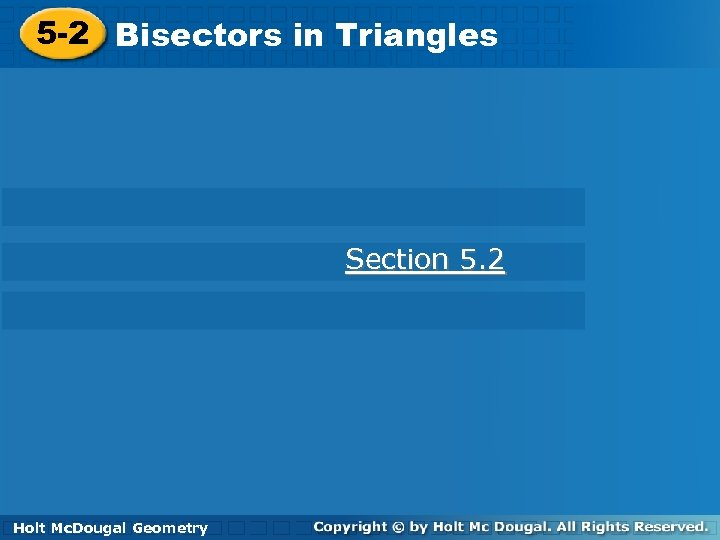5 -2 Bisectors in Triangles Section 5. 2 Holt Geometry Holt Mc. Dougal Geometry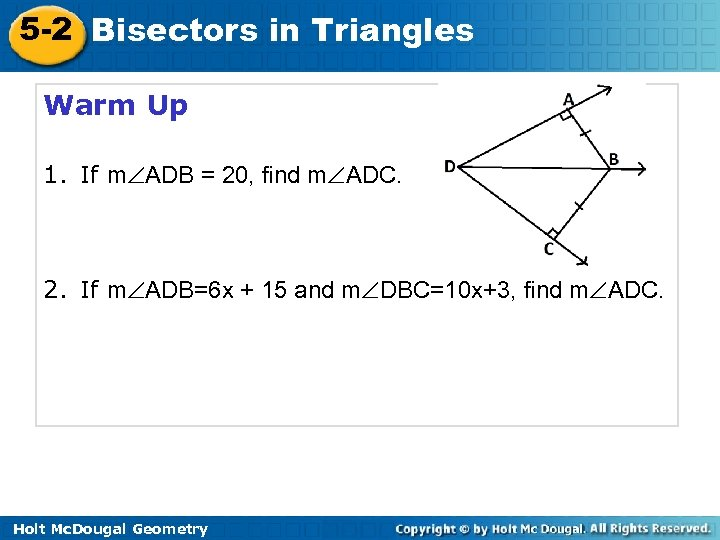5 -2 Bisectors in Triangles Warm Up 1. If m ADB = 20, find m ADC. 2. If m ADB=6 x + 15 and m DBC=10 x+3, find m ADC. Holt Mc. Dougal Geometry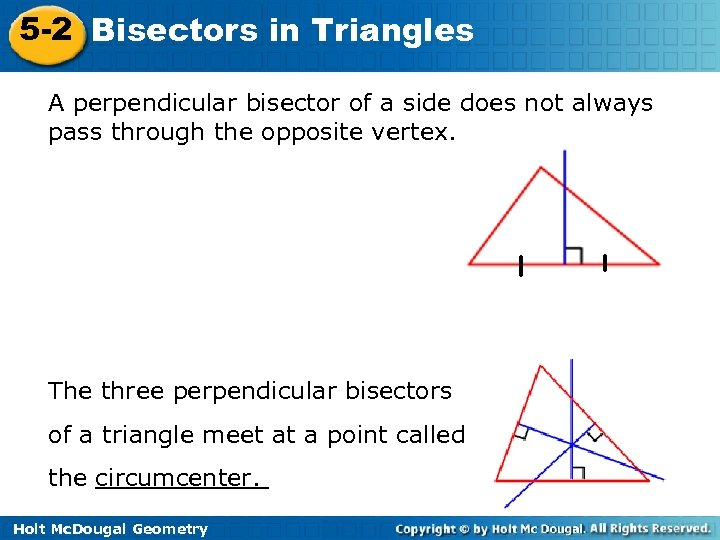5 -2 Bisectors in Triangles A perpendicular bisector of a side does not always pass through the opposite vertex. The three perpendicular bisectors of a triangle meet at a point called the circumcenter. Holt Mc. Dougal Geometry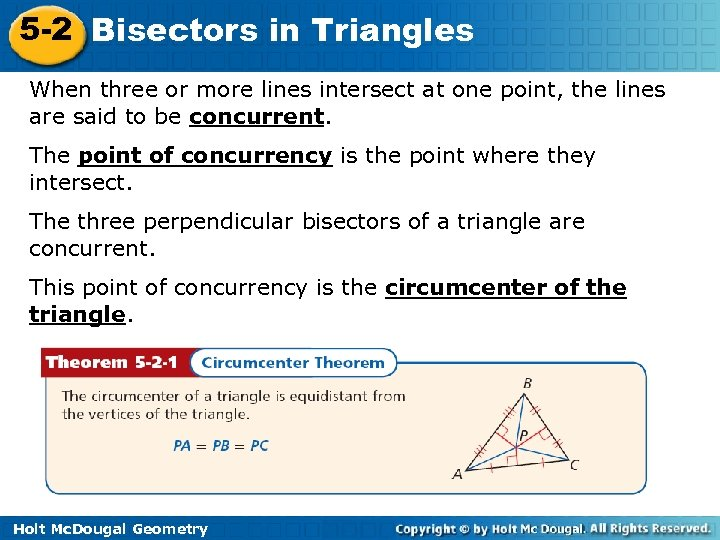5 -2 Bisectors in Triangles When three or more lines intersect at one point, the lines are said to be concurrent. The point of concurrency is the point where they intersect. The three perpendicular bisectors of a triangle are concurrent. This point of concurrency is the circumcenter of the triangle. Holt Mc. Dougal Geometry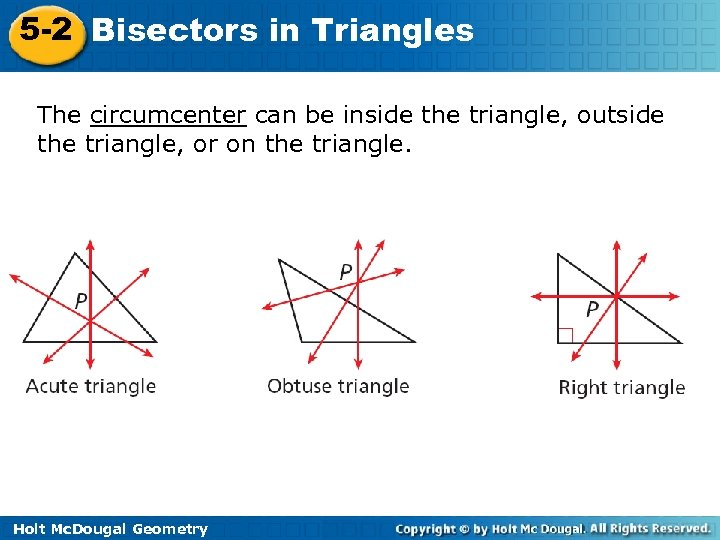5 -2 Bisectors in Triangles The circumcenter can be inside the triangle, outside the triangle, or on the triangle. Holt Mc. Dougal Geometry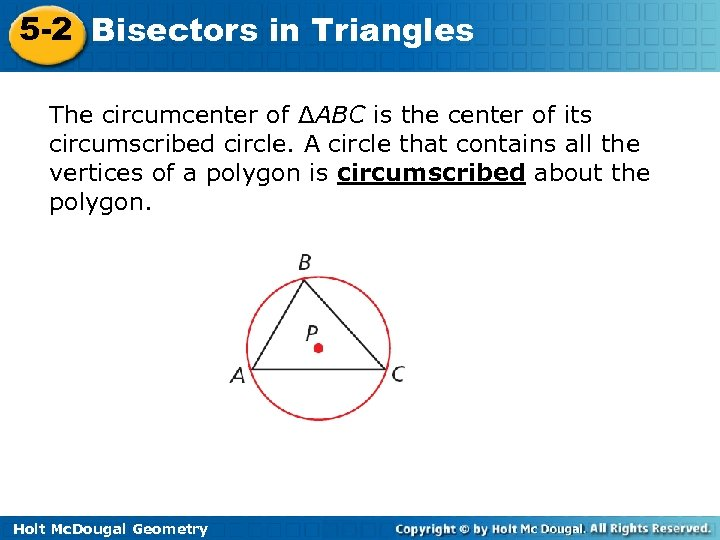5 -2 Bisectors in Triangles The circumcenter of ΔABC is the center of its circumscribed circle. A circle that contains all the vertices of a polygon is circumscribed about the polygon. Holt Mc. Dougal Geometry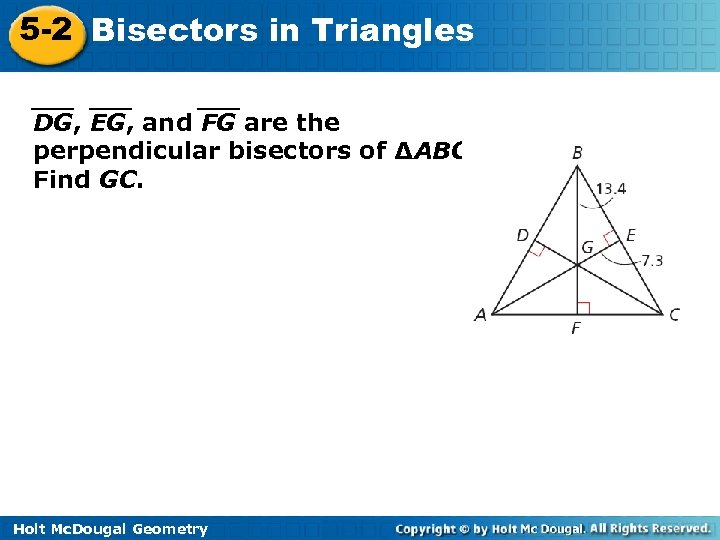5 -2 Bisectors in Triangles DG, EG, and FG are the perpendicular bisectors of ∆ABC. Find GC. Holt Mc. Dougal Geometry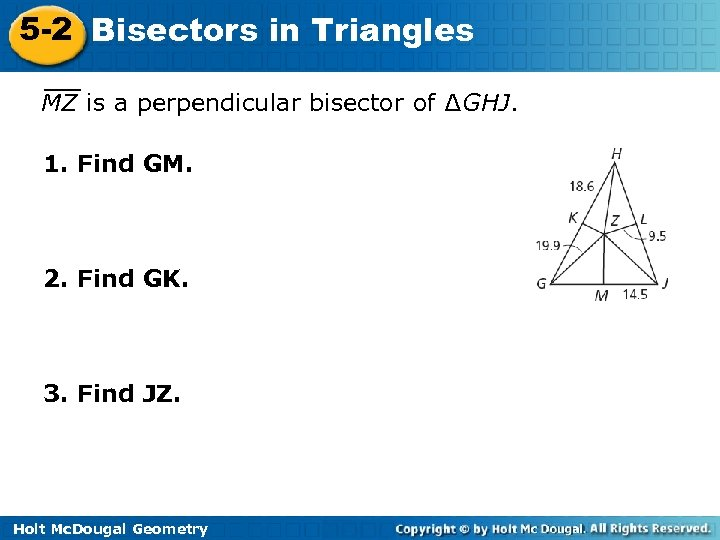5 -2 Bisectors in Triangles MZ is a perpendicular bisector of ∆GHJ. 1. Find GM. 2. Find GK. 3. Find JZ. Holt Mc. Dougal Geometry5 -2 Bisectors in Triangles Find the circumcenter of ∆HJK with vertices H(0, 0), J(10, 0), and K(0, 6). Find the circumcenter of ∆GOH with vertices G(0, – 9), O(0, 0), and H(8, 0). Holt Mc. Dougal Geometry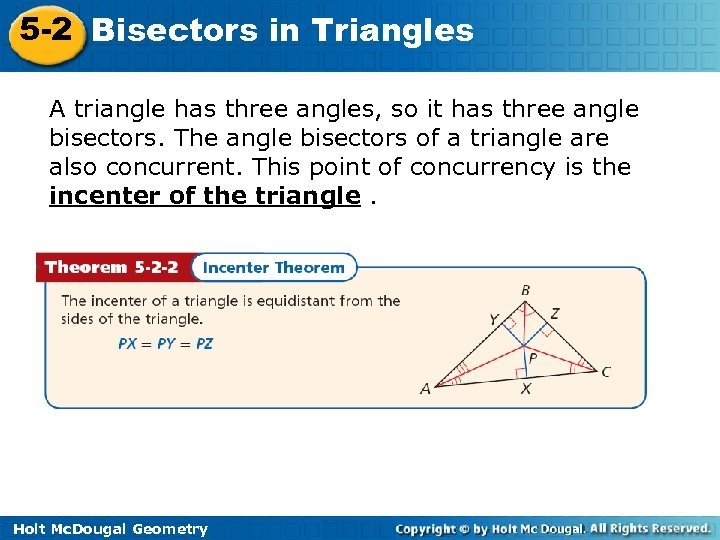5 -2 Bisectors in Triangles A triangle has three angles, so it has three angle bisectors. The angle bisectors of a triangle are also concurrent. This point of concurrency is the incenter of the triangle. Holt Mc. Dougal Geometry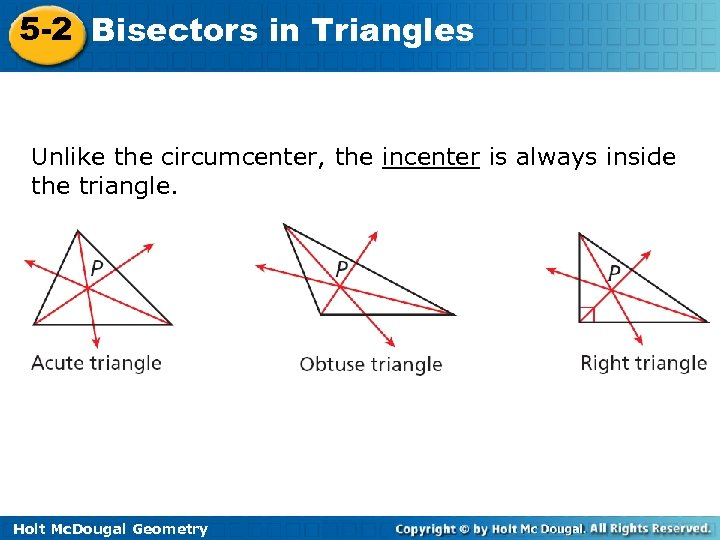5 -2 Bisectors in Triangles Unlike the circumcenter, the incenter is always inside the triangle. Holt Mc. Dougal Geometry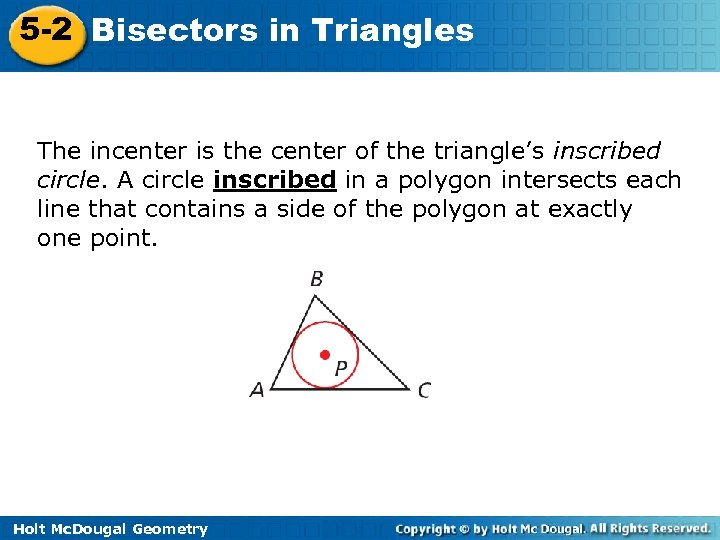5 -2 Bisectors in Triangles The incenter is the center of the triangle’s inscribed circle. A circle inscribed in a polygon intersects each line that contains a side of the polygon at exactly one point. Holt Mc. Dougal Geometry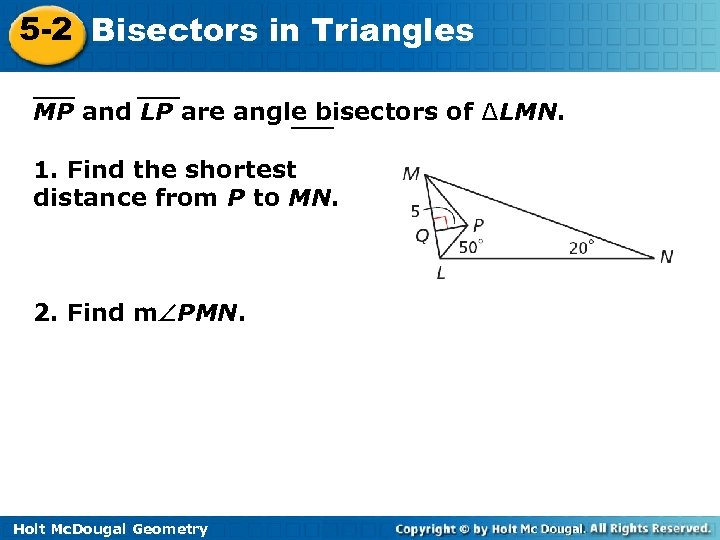5 -2 Bisectors in Triangles MP and LP are angle bisectors of ∆LMN. 1. Find the shortest distance from P to MN. 2. Find m PMN. Holt Mc. Dougal Geometry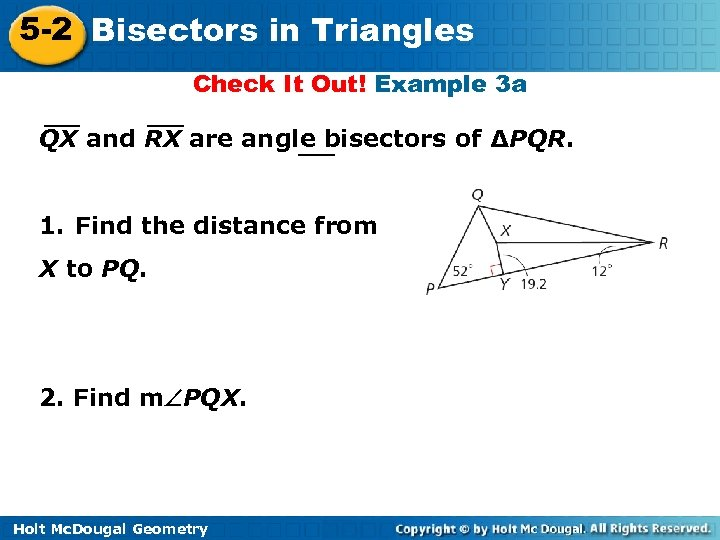5 -2 Bisectors in Triangles Check It Out! Example 3 a QX and RX are angle bisectors of ΔPQR. 1. Find the distance from X to PQ. 2. Find m PQX. Holt Mc. Dougal Geometry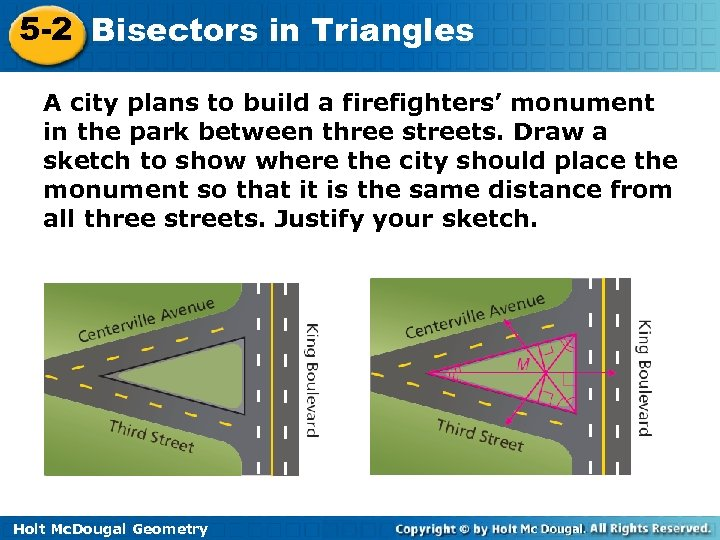5 -2 Bisectors in Triangles A city plans to build a firefighters’ monument in the park between three streets. Draw a sketch to show where the city should place the monument so that it is the same distance from all three streets. Justify your sketch. Holt Mc. Dougal Geometry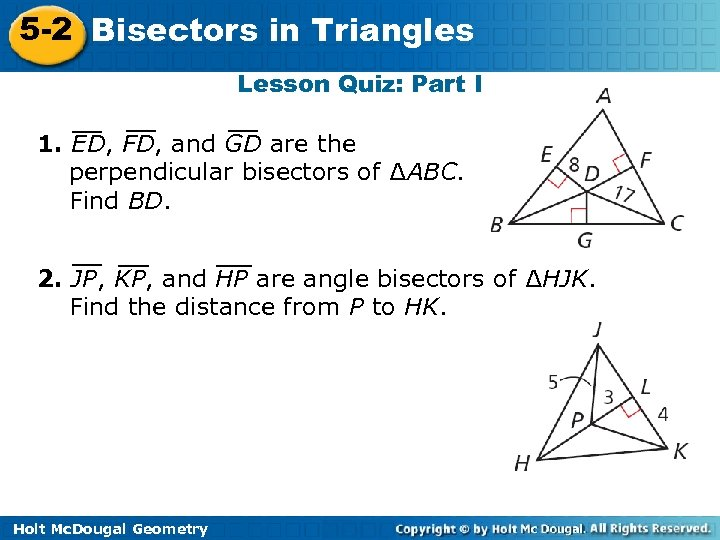5 -2 Bisectors in Triangles Lesson Quiz: Part I 1. ED, FD, and GD are the perpendicular bisectors of ∆ABC. Find BD. 2. JP, KP, and HP are angle bisectors of ∆HJK. Find the distance from P to HK. Holt Mc. Dougal Geometry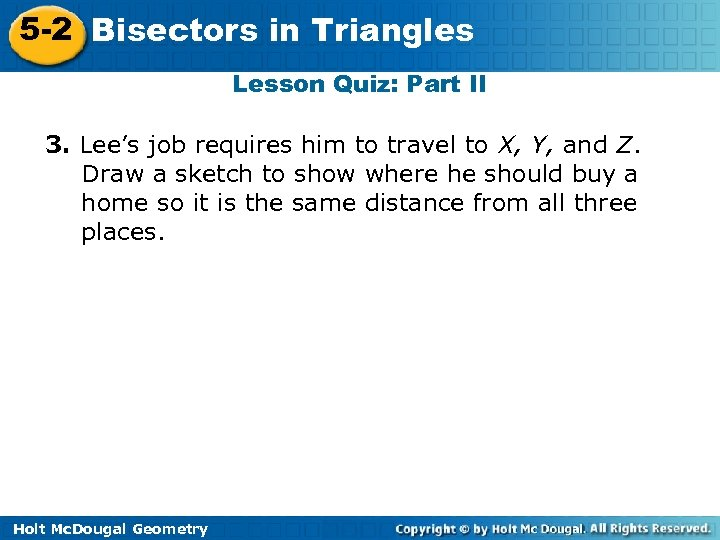5 -2 Bisectors in Triangles Lesson Quiz: Part II 3. Lee’s job requires him to travel to X, Y, and Z. Draw a sketch to show where he should buy a home so it is the same distance from all three places. Holt Mc. Dougal Geometry